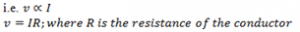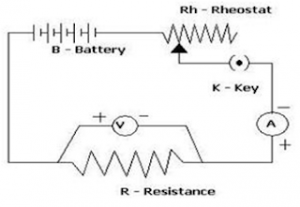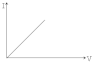# Ohm’s Law

It states, the current passing through any conductor is proportional to the potential difference applied across the conductor provided that physical condition (mechanical strain, temperature) of the conductor remains unchanged.Verification of Ohm’s LawOhm’s law can be verified by using a simple circuit as shown in fig. aside. A resistance R is connected in a series to a battery, ammeter and a rheostat. A voltmeter is connected in parallel across a fixed resistance R. When the key is closed, I current flows in the circuit and voltmeter and ammeter shows reading. By adjusting rheostat at different position different value of V and A are obtained. These values are noted and plotted on a graph paper and the graph shown below is obtained.Here a straight line passing through origin is obtained. This graph shows V is proportions to I which is Ohm’s Law.
Hence verified.